## The Delta Function Model of a Crystal *

The Kronig-Penny model of a solid crystal contains an infinite array of repulsive delta functions.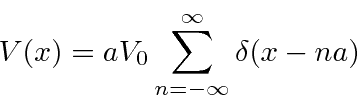Our states will have positive energy.

This potential has a new symmetry, that a translation by the lattice spacing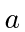leaves the problem unchanged. The probability distributions must therefore have this symmetry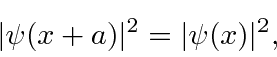which means that the wave function differs by a phase at most.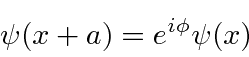The general solution in the regionisBy matching the boundary conditions and requiring that the probability be periodic, we derive a constraint on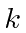similar to the quantized energies for bound states.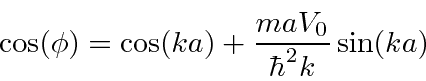Since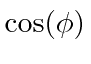can only take on values between -1 and 1, there are allowed bands ofand gaps between those bands.

The graph below showsas a function of. If this is not between -1 and 1, there is no solution, that value ofand the corresponding energy are not allowed.This energy band phenomenon is found in solids. Solids with partially filled bands are conductors. Solids with filled bands are insulators. Semiconductors have a small number of charge carriers (or holes) in a band.

Jim Branson 2013-04-22